In this page we have NCERT book Solutions for Class 9th Maths:Quadrilaterals for EXERCISE 8.1 . Hope you like them and do not forget to like , social share and comment at the end of the page.
We must revise these notes before proceding with the questions

## Different Criterion for Congruence of the triangles

### SAS Congurence (AXIOM)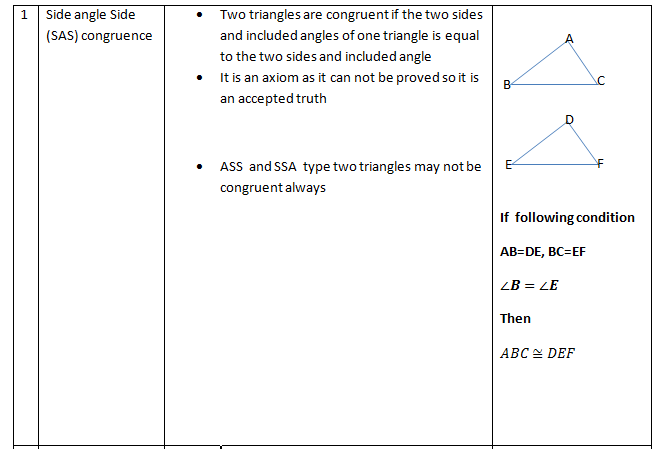### ASA Congurence (Theorem)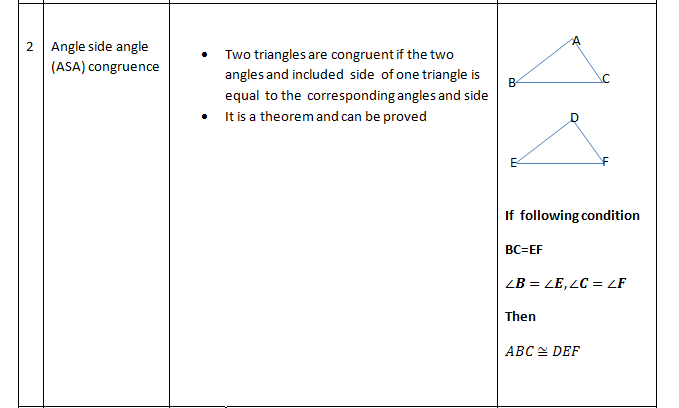### AAS Congurence (Theorem)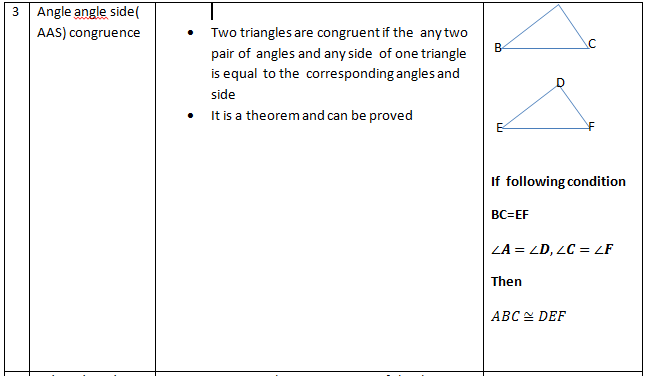### SSS Congurence (Theorem)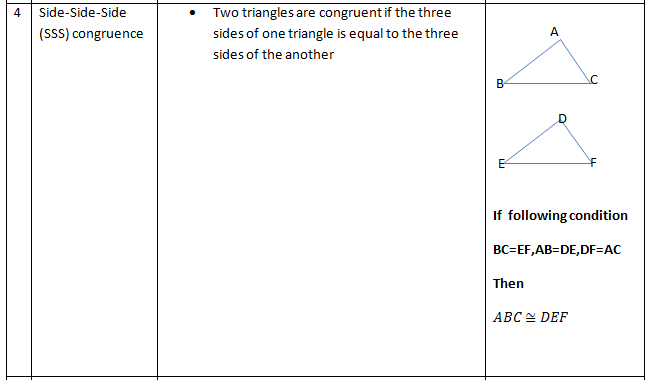### RHS Congurence (Theorem)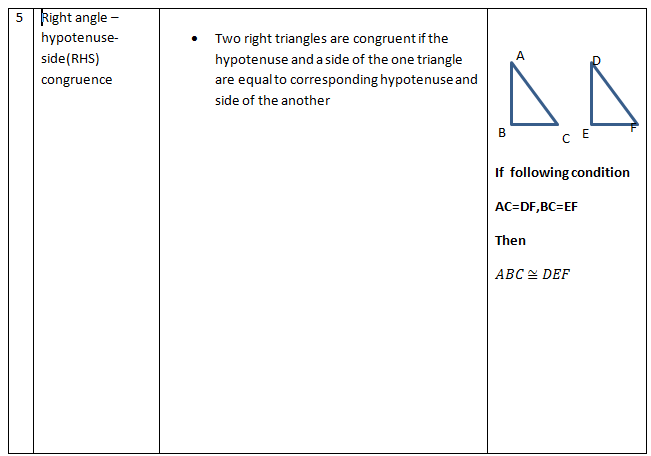Caveat
AAA( Angle Angle Angle) is not the right condition to Prove congurence.

Question 1
The angles of quadrilateral are in the ratio 3 : 5 : 9 : 13. Find all the angles of the Quadrilateral
Solution:
Let the common ratio between the angles be y. Therefore, the angles will be 3y, 5y, 9y, and 13y respectively.
As the sum of all interior angles of a quadrilateral is 3600
3y + 5y + 9y + 13y = 3600
30y= 3600
y = 120
Hence, the angles are
3y=36
5y = 5 × 12 = 600
9y = 9 × 12 = 1080
13y = 13 × 12 = 1560

Question 2
If the diagonals of a parallelogram are equal, then show that it is a rectangle
Solution:
Let ABCD is a parallelogram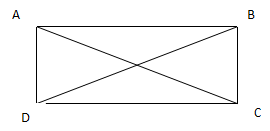Given
AC=BD and AD=BC and CD=AB (in parallelogram opposite side are equal)
To Prove:
Show that it is a rectangle
Proof
In Δ ADC and Δ BCD
CD =CD
AC=BD
So by SSS congruence
So ∠ADC=∠BCD by CPCT (corresponding parts of the two congruent triangles)
So ∠BCD=90
So all the angles A, B, C, D are 900 .Hence rectangles

Question 3
Show that if the diagonals of a quadrilateral bisect each other at right angles, then it is a rhombus.
Solution:
Given
Let ABCD be the quadrilateral and AC and BD are diagonal which bisect at right angles
OA = OC, OB = OD and AOB = BOC = COD = AOD = 90º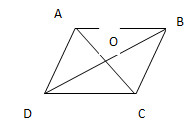To Prove: ABCD is rhombus
Proof:
Now, in ΔAOD and Δ COD
OA = OC (Diagonal bisects each other)
∠AOD =∠COD (given)
OD = OD (common)
So by SAS congruence rule
ΔAOD ≅ ΔCOD AD = CD(by CPCT) (i)
Similarly we can prove that
AD = AB and CD = BC (ii)
From equations (i) and (ii), we can say that
AB = BC = CD = AD
Since all sides are equal, it is a rhombus

Question 4
Show that the diagonals of a square are equal and bisect each other at right angles.
Solution:
Let ABCD is a square whose diagonal BD and AC intersect art O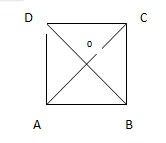GivenTo prove: Diagonal are equal and bisects each other at right angle
OA=OC
OD=OB
AC=BD
Proof:
In ΔABC andΔDCB,
AB = DC ( From Sides of a square are equal to each other)
∠ABC =∠DCB (All interior angles are of 90)
BC = CB (Common side)
So by SAS congruence
ΔABC≅ΔDCB
AC = DB (By CPCT)
Hence, the diagonals of a square are equal in length.
In ΔAOB and ΔCOD,
∠AOB =∠COD (Vertically opposite angles)
∠ABO =∠CDO (Alternate interior angles)
AB = CD (Sides of a square are always equal)
By AAS congruence rule
ΔAOB ≅ ΔCOD
∴AO = CO and OB = OD (By CPCT)
Hence, the diagonals of a square bisect each other.

In ΔAOB and ΔCOB,
As we had proved that diagonals bisect each other, therefore,
AO = CO
AB = CB ( we know that Sides of a square are equal)
BO = BO (Common)
By SSS congruency
ΔAOB ≅ ΔCOB

∴∠AOB =∠COB (By CPCT)
However,∠AOB +∠COB = 1800 (Linear pair)
2∠AOB = 1800
∠AOB = 900
Hence, the diagonals of a square bisect each other at right angles.

Question 5
Show that if the diagonals of a quadrilateral are equal and bisect each other at right angles, then it is a square
Solution:
Let ABCD is a quadrilateral whose diagonal BD and AC bisect each other at right angle at O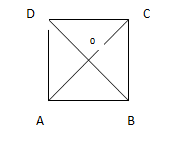Given
AO=OC, BO=OD, AC=BD
∠AOB=∠COD
To Prove: ABCD is a square
Proof:
In ΔAOB and ΔCOD
AO=CO (Given)
∠AOB=∠COD (Each given equal to 90o)
BO=DO (Given)
So by SAS congruence rule,
ΔAOB ≅ ΔCOD.
Therefore
∠OBA=∠ODC (by CPCT)
But, these are alternate interior angles which means that AB?CD. (i)
Similarly, we can prove that BC?AD (ii)
From (1) and (2), we can say that quadrilateral ABCD is a parallelogram. Hence, we have AB=CD and BC=AD because opposite sides of a parallelogram are equal. (iii)

Now, in ΔAOB and ΔAOD
AO=AO (Common)
∠AOB=∠AOD (Each given equal to 90o)
OB=OD (Given)
So by SAS congruence rule
ΔAOB ≅ ΔAOD
Therefore

In ΔACD and ΔBDC
AC=BD (Given)
AD=BC (Proved above in (1) )
CD=DC (Common)
So by SSS congruence rule
ΔACD ≅ ΔBDC
Therefore
But, we also have
∠ADC + ∠BCD= 180o (Co-Interior angles) (vi)
From (5) and (6), we can say that

From (iii), (iv) -> we can say that ABCD is a parallelogram having all the sides equal.
(vii) -> we have showed that it's one angle is equal to 90owhich is enough to consider it a square. Therefore, ABCD is a square.

Question 6
Diagonal AC of a parallelogram ABCD bisects ∠A (see the given figure). Show that
(i) It bisects ∠C also,
(ii) ABCD is a rhombus.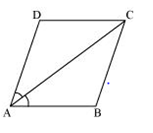Solution:
(i)
ABCD is a parallelogram and AC bisect angle A
∠DAC =∠BAC

To Prove: AC bisects ∠C
Proof:
As ABCD is a parallelogram
∠DAC =∠BCA (Alternate interior angles) ... (i)
And ∠BAC =∠DCA (Alternate interior angles) ... (ii)
But it is given that AC bisects ∠A.
∠DAC =∠BAC ... (iii)
From equations (i), (ii) and (iii), we have
∠DAC =∠BCA =∠BAC =∠DCA ... (iv)
=> ∠DCA =∠BCA
Hence, AC bisects ∠C.

(ii)
To Prove: ABCD is a rhombus
Proof :
From equation (iv), we have
∠DAC =∠DCA DA = DC (we know that side opposite to equal angles are equal)
But DA = BC and AB = CD (opposite sides of parallelogram are equal)
AB = BC = CD = DA
Hence, ABCD is rhombus

Question 7
ABCD is a rhombus. Show that diagonal AC bisects ∠A as well as ∠C and diagonal BD bisects ∠B as well as ∠D.
Solution
Given: ABCD is a rhombus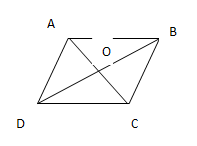To Prove: Diagonal BD bisect angle B and D
Diagonal AC bisect angle A and C
Proof:
Let us join AC.
In ΔABC,
BC = AB (Sides of a rhombus are equal to each other)
∴∠BAC =∠BCA (Angles opposite to equal sides of a triangle are equal)
However,∠BAC =∠DCA (Alternate interior angles for parallel lines AB and CD)
∠BCA=∠DCA
Therefore, AC bisects ∠C.
Also,∠BCA=∠DAC (Alternate interior angles for || lines BC and DA)
∠BAC =∠DAC
Therefore, AC bisects ∠A.
Similarly, it can be proved that BD bisects ∠B and ∠D as well.

Question 8
ABCD is a rectangle in which diagonal AC bisects ∠A as well as ∠C. Show that:
(i)ABCD is a square
(ii) Diagonal BD bisects ∠ B as well as ∠ D
Solution
Given
ABCD is a rectangle and ∠DAC=∠BAC and ∠DCA=∠BCA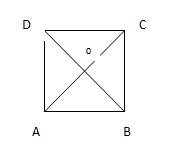(i)
To Prove: ABCD is a square
Proof:
AC=AC (Common)
∠DCA=∠BCA (Given)
Therefore, by ASA congruence rule
From (1) and (2), we can say that ABCD is a rectangle having all the sides equal. It means that ABCD is a square.

(ii)
To prove diagonal BD bisects ∠B as well as ∠D.
In solution (i), we have showed that ABCD is a square.
Now in ΔCBD and ΔABD
BC=BA (Sides of square are equal)
BD=BD (Common)
CD=AD (Sides of square are equal)
So by SSS congruence rule
ΔCBD ≅ ΔABD
∠CBD=∠ABD (by CPCT) (3)
From (3) and (4), we can say that BD bisects ∠B as well as ∠D.

Latest Articles
Synthetic Fibres and Plastics Class 8 Practice questions

Class 8 science chapter 5 extra questions and Answers

Mass Calculator

3 Fraction calculator

Garbage in Garbage out Extra Questions7